hasChangedFrom

Detect change in data from specified value

Description

example

tf = hasChangedFrom(data_name,value) returns 1 (true) if the value of data_name was equal to the specified value at the beginning of the previous time step and is a different value at the beginning of the current time step. Otherwise, the operator returns 0 (false).

The argument data_name can be:

• A scalar variable.

• A matrix or an element of a matrix.

• If data_name is a matrix, the operator returns true when it detects a change in any element of data_name and the previous value of data_name was equal to value.

• Index elements of a matrix by using numbers or expressions that evaluate to a constant integer. See Supported Operations for Vectors and Matrices.

• A structure or a field in a structure.

• If data_name is a structure, the change detection operator returns true when it detects a change in any element of data_name and the previous value of data_name was equal to value.

• Index fields in a structure by using dot notation. See Index and Assign Values to Stateflow Structures.

• Any valid combination of structure fields or matrix elements.

The argument data_name cannot be a nontrivial expression or a custom code variable.

Note

Standalone charts in MATLAB® do not support change detection on an element of a matrix or a field in a structure.

The argument value can be any expression that resolves to a value that is comparable with data_name:

• If data_name is a scalar, then value must resolve to a scalar value.

• If data_name is a matrix, then value must resolve to a matrix value with the same dimensions as data_name.

Alternatively, in a chart that uses C as the action language, value can resolve to a scalar value. The chart uses scalar expansion to compare data_name to a matrix whose elements are all equal to the value specified by value. See Assign Values to All Elements of a Matrix.

• If data_name is a structure, then value must resolve to a structure value whose field specification matches data_name exactly.

Examples

expand all

Transition out of state if the previous value of the matrix M was equal to matrixValue and any element of M has changed value since the last time step or input event.

[hasChangedFrom(M,matrixValue)]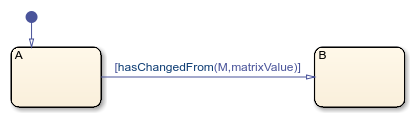Transition out of state if the element in row 1 and column 3 of the matrix M has changed from the value 7 since the last time step or input event.

In charts that use MATLAB as the action language, use:

[hasChangedFrom(M(1,3),7)]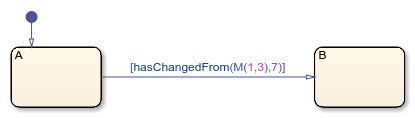In charts that use C as the action language, use:

[hasChangedFrom(M,7)]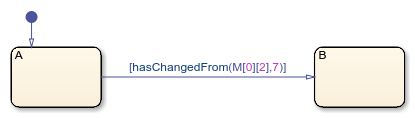Transition out of state if the previous value of the structure struct was equal to structValue and any field of struct has changed value since the last time step or input event.

[hasChangedFrom(struct,structValue)]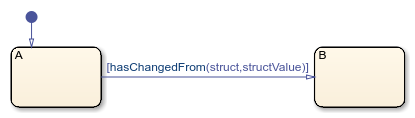Transition out of state if the field struct.field has changed from the value 5 since the last time step or input event.

[hasChangedFrom(struct.field,5)]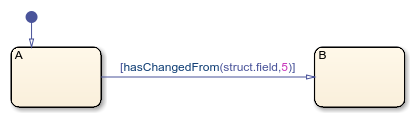Tips

• If multiple input events occur in the same time step, the hasChangedFrom operator can detect changes in data value between input events.

• If the chart writes to the data object but does not change the data value, the hasChangedFrom operator returns false.

• The type of Stateflow® chart determines the scope of the data supported by the change detection operators:

• Standalone Stateflow charts in MATLAB: Local only

• In Simulink® models, charts that use MATLAB as the action language: Input only

• In Simulink models, charts that use C as the action language: Input, Output, Local, or Data Store Memory

• In a standalone chart in MATLAB, a change detection operator can detect changes in data specified in a call to the step function because these changes occur before the start of the current time step. For example, if x is equal to zero, the expression hasChangedFrom(x,0) returns true when you execute the chart ch with the command:

step(ch,'x',1);
In contrast, a change detection operator cannot detect changes in data caused by assignments in state or transition actions in the same time step. Instead, the operator detects the change in value at the start of the next time step.

• In a chart in a Simulink model, if you enable the chart option Initialize Outputs Every Time Chart Wakes Up, using an output as the argument of the hasChanged operator always returns false. For more information, see Initialize outputs every time chart wakes up.

• When row-major array layout is enabled in charts that use hasChangedFrom, code generation produces an error. Before generating code in charts that use hasChangedFrom, enable column-major array layout. See Select Array Layout for Matrices in Generated Code.

Introduced in R2007a

Stateflow DocumentationGet trial now